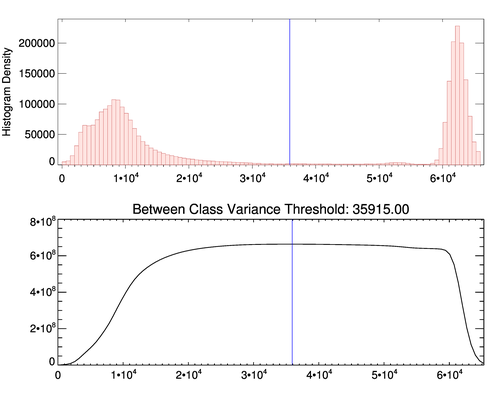# Coyote Graphics Routines

## Date: Fri Mar 27 12:14:20 2015

single page | use frames     summary     class     fields     routine details     file attributes

# cgotsu_threshold.pro

## top cgOTSU_THRESHOLD

Utility

`result = cgOTSU_THRESHOLD(data [, BINSIZE=BINSIZE] [, /EXAMPLE] [, HISTDATA=HISTDATA] [, /L64] [, LOCATIONS=LOCATIONS] [, MAX=MAX] [, MIN=MIN] [, MISSING=MISSING] [, /NAN] [, NBINS=integer] [, OMAX=OMAX] [, OMIN=OMIN] [, /PLOTIT] [, REVERSE_INDICES=REVERSE_INDICES])`

The purpose of this function is to find an optimal threshold for separating a bimodal distribution of pixels in an image histogram. The algorithm used is the "faster approach" algorithm explained on this web page.### Return value

The optimal threshold that separates two populations of pixels is returned.

### Parameters

data in required

The data from which the histogram is created.

### Keywords

BINSIZE in optional

The binsize of the histogram. By default, Scott's Choice of bin size for histograms is used:

``` binsize = (3.5 * StdDev(data)) / N_Elements(data)^(0.3333) ```
unless the data is byte type. Then a BINSIZE of 1 is used by default

If BINSIZE in not defined, and NBINS is defined, the BINSIZE is calcuated as:

``` binsize = (Max(dataToHistogram) - Min(dataToHistogram)) / (NBINS -1) ```
While it is pointed out in the HISTOGRAM documentation, it is extremely important that the BINSIZE be of the same data type as the data you are going to calculate the histogram of. If it is not VERY strange things can happen. I've tried to protect you from most of the bad things, but I don't have a high confidence level that I have done it for every situation. If you see something that "just don't look right", I would check first to see if your data types match. That might solve all your problems.

EXAMPLE in optional type=boolean default=0

Set this keyword if you wish to use the example data from the reference documentation.

HISTDATA out optional

The output value of the internal HISTOGRAM command.

L64 in optional type=boolean default=0

If set, the return value of HISTOGRAM are 64-bit integers, rather than the default 32-bit integers. Set by default for data types greater than or equal to 12.

LOCATIONS out optional

Starting locations of each bin. (See the HISTOGRAM documentation for details.)

MAX in optional

The maximum value to use in calculating input histogram. Equivalent to the MAX keyword in the HISTOGRAM documentation.

MIN in optional

The minimum value to use in calculating input histogram. Equivalent to the MIN keyword in the HISTOGRAM documentation.

MISSING in optional

The value that should be represented as "missing" and not used in the histogram. Be aware that if the input data is not of type "float" or "double" that the input data will be converted to floating point prior to calculating the histogram.

NAN in optional type=boolean default=0

If set, ignore NAN values in calculating and plotting histogram.

NBINS in optional type=integer

The number of output bins in the histogram. Meaning is slightly different from meaning in the HISTOGRAM command. Used only to calculate BINSIZE when BINSIZE is not specified. In this case, binsize = rangeofData/(nbins-1).

OMAX out optional

The maximum output value used to construct the histogram. (See HISTOGRAM documentation.)

OMIN out optional

The minimum output value used to construct the histogram. (See HISTOGRAM documentation.)

PLOTIT in optional type=boolean default=0

If this keyword is set, a histogram of the data is plotted along with a plot of the between class variance to show the selected threshold.

REVERSE_INDICES out optional

The list of reverse indices returned from the HISTOGRAM command. (See HISTOGRAM documentation.)

### Examples

Set the `Example` keyword to use the data from the reference page.

### Author information

Author:

FANNING SOFTWARE CONSULTING:

``` David W. Fanning 1645 Sheely Drive Fort Collins, CO 80526 USA Phone: 970-221-0438 E-mail: david@idlcoyote.com Coyote's Guide to IDL Programming: http://www.idlcoyote.com ```

``` Written by: David W. Fanning, 13 November 2012, from a program named OTSU_THRESHOLD by Carl Salvaggio and modified by Gianguido Cianci. The OTSU_THRESHOLD algorithm used previously made many assumptions about the data. The algorithm used here has been completely rewritten to comply with the values in the reference page and to avoid making assumptions about the data used to create the histogram. 21 November 2012. DWF. Modified to set L64 keyword if data type GE 14 (suggested by user). 22 November 2012. DWF. Modified the threshold value to use DIndGen instead of IndGen, which was causing incorrect results with integer data. 24 November 2012. DWF. ```